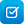# Search results

Year level
Resource type
Learning area

## Refine by topic

Main topic Specific topic Related topic
Listed under:  Angles### Trigonometry: finding angles

Look at an explanation of the trigonometric ratios: sine, cosine and tangent. Adjust the angles of a right-angled triangle within a unit circle (radius of one unit). Set the cosine, sine and tangent values of angles from 0–90 degrees. Identify the angle for each of the values. This learning object is one in a series of ...### TIMES Module 22: Measurement and Geometry: introductory trigonometry - teacher guide

This is a 20-page guide for teachers containing an introduction to the three basic trigonometric ratios. A history of trigonometry concludes the module.### Trig degrees

Use a unit circle tool to explore sine, cosine and tangent values in different quadrants. Determine the values for angles between 0° and 360°. Observe the symmetry and patterns of the functions to predict the behaviour of the graphs and the values for the functions in different quadrants. Observe the graphs animate and ...### Trig degrees: tangent to 360

Use a unit circle tool to explore tangent values in different quadrants. Determine the tan Ø values for angles between 0° and 360°. Observe the symmetry and patterns of the functions to predict the behaviour of tan Ø in different quadrants. Observe the graph animate and visually connect it with the unit circle tool and ...Use a unit circle tool to explore sine, cosine and tangent values in different quadrants. Determine the values for angles between 0 and 2π radians. Observe the symmetry and patterns of the functions to predict the behaviour of the graphs and the values for the functions in different quadrants. Observe the graphs animate ...### Trigonometry: assessment

Test your understanding of angles and their sine, cosine and tangent values. Use a unit circle tool to find sine, cosine and tangent values in different quadrants. Use the tool to find the angle when given sine, cosine or tangent values. Determine the values for angles between 0° and 360°. Solve problems by using the unit ...### Exploring relationships of angles

Explore angles formed by a transversal line intersecting parallel lines. Look at illustrations showing pairs of angles: vertically opposite, corresponding and alternate angles. Name pairs of angles to score points and help a monkey drive to the supermarket to buy food.### EagleCat: trig-G

Explore the graphs of trigonometric equations in the form: (a) y = a sin[n(x - h)] + k, (b) y = a cos[n(x - h)] + k and (c) y = a tan[n(x - h)] + k. Use sliders or enter values to dilate, reflect and translate the basic trigonometric equations y = sin(x), y = cos(x) and y = tan(x), and observe the changes in the amplitude, ...### Trigonometry: sine

Look at an explanation of the trigonometric ratios: sine, cosine and tangent. Adjust the angles of a right-angled triangle within a unit circle (radius of one unit). Find corresponding sides in similar triangles and find relationships between ratios. Identify the sine ratio and enter the sine values of angles from 0–90 ...### TIMES Module 9: Measurement and Geometry: introduction to plane geometry - teacher guide

This is a 16-page guide for teachers. It provides an introduction to the initial ideas of plane geometry. Points and lines are introduced as fundamental objects in the study of geometry. Angles and parallelism are the initial areas of attention in a more formal approach to geometry that occurs from year 7.### TIMES Module 26: Measurement and Geometry: circle geometry - teacher guide

This is a 44-page guide for teachers. It contains theorems on chords, arcs, angles and secants associated with circles.### Angles (estimating angle size)

This is a six-page HTML resource about solving problems involving estimating angle size. It contains one video and three questions, two of which are interactive. The resource discusses and explains solving problems to reinforce students' understanding.### Angle Types

An animated tutorial about types of angles followed by an interactive quiz.### Right angle or acute angle?

What's the difference between a right angle and an acute angle? Find out!### Trig degrees: sine to 360

Use a unit circle tool to explore sine values in different quadrants. Determine the sin Ø values for angles between 0° and 360°. Observe the symmetry and patterns of the functions to predict the behaviour of sin Ø in different quadrants. Observe the graph animate and visually connect it with the unit circle tool and table ...### Trig degrees: cosine to 360

Use a unit circle tool to explore cosine values in different quadrants. Determine the cos Ø values for angles between 0° and 360°. Observe the symmetry and patterns of the functions to predict the behaviour of cos Ø in different quadrants. Observe the graph animate and visually connect it with the unit circle tool and table ...Use a unit circle tool to explore sine values in different quadrants. Determine the sin Ø values for angles between 0 and 2π radians. Observe the symmetry and patterns of the functions to predict the behaviour of sin Ø in different quadrants. Observe the graphs animate and visually connect them with the unit circle tool ...Use a unit circle tool to explore cosine values in different quadrants. Determine the cos Ø values for angles between 0 and 2π radians. Observe the symmetry and patterns of the functions to predict the behaviour of cos Ø in different quadrants. Observe the graphs animate and visually connect them with the unit circle tool ...